# 漫谈C语言指针（三）C内存与指针：

# 二级指针

``````int a =5;
int *p1 = &a;
int **p2 = &p1;

## 在函数内部修改函数外部一个指针的指向：

``````void secondPointer(int **a) {
int *p = static_cast<int *>(malloc(sizeof(int)));//用malloc保证离开函数后内存不会释放
*p = 5;
*a = p;//改变a函数外传入的二级指针指向的指针的指向
printf("secondPointer *a %#X\n", *a);
}

int main() {
int i = 10;
int *a = &i;
printf("before secondPointer a %#X\n", a);//改变a之前的指向之前a的指向地址

secondPointer(&a);
printf("after secondPointer a %#X\n", a);//改变a之后的指向之前a的指向地址
printf("after secondPointer *a %d\n", *a);//改变a之前的指向之前a的指向地址
}

``````before secondPointer a 0X62FE1C
secondPointer *a 0XDB1C40
after secondPointer a 0XDB1C40
after secondPointer *a 5

## 二维数组

int a = { {0, 1, 2, 3}, {4, 5, 6, 7}, {8, 9, 10, 11} };

``````0   1   2   3
4   5   6   7
8   9  10  11

C语言中的二维数组是按行排列的，也就是先存放 a 行，再存放 a 行，最后存放 a 行；每行中的 4 个元素也是依次存放。数组 a 为 int 类型，每个元素占用 4 个字节（假设当前环境int占用4字节），整个数组共占用 4×(3×4) = 48 个字节。

``````int (*p) = &a;//数组指针p指向数组a

``````int a[] = {1,2,3,4,5,6};
int (*p) = &a;
printf("secondPointer sizeof(int) %d\n", sizeof(int));
printf("secondPointer p %#X\n", p);
printf("secondPointer p+1 %#X\n", p+1);

C语言允许把一个二维数组分解成多个一维数组来处理。对于数组 a，它可以分解成三个一维数组，即 a、a、a。每一个一维数组又包含了 4 个元素，例如 a 包含 a、a、a、a。

`````` int a = { {0, 1, 2, 3}, {4, 5, 6, 7}, {8, 9, 10, 11} };
printf("sizeof(*(a)):%d\n", sizeof(*a));//求出a指向的数据的大小
printf("a:%d\n", a);
printf("a+1%:d\n", a+1);//求a的步长（其实和sizeof(*a)一样大）

printf("sizeof(*a):%d\n", sizeof(*a));//求出a指向的数据的大小
printf("a:%d\n", a);
printf("a+1:%d\n", a+1);//求a的步长（其实和sizeof(*a)一样大）

printf("&a:%d\n", &a);//要记得数组名在&符号的时候不会转化为指针，所以是对数组取地址
printf("&a+1:%d\n", &a+1);//看下&a的步长

``````int a = { {0, 1, 2, 3}, {4, 5, 6, 7}, {8, 9, 10, 11} };

for(int i=0; i<3; i++){
for(int j=0; j<4; j++)
printf("value :%d\n",*(*(a+i)+j));
}

# 指针数组

``````int (*p);

``````int *p;

``````int a = 1, b = 2, c = 3;
//定义一个指针数组
int *arr = {&a, &b, &c};//创建一个指针数组

``````int a = 1, b = 2, c = 3;
//定义一个指针数组
int *arr = {&a, &b, &c};//创建一个指针数组
printf("%d, %d, %d\n", **(arr+0), **(arr+1), **(arr+2));//因为数组名arr转化为二级指针，所以要加2个**来取值

`````` char *str = {
"man",
"woman",
"pig"
};

``````printf("%c\n", *(*(str+1)+3));//*（str+1）是得到"woman"的首地址，*(*(str+1)+3))是"woman"的首地址偏移3个char之后，再取值，即‘a’。

# 函数指针

``````int(*p)(int, int);

``````/**
* 加法运算
* @param a
* @param b
* @return
*/
int plus(int a,int b){
return a+b;
}

/**
* 减法运算
* @param a
* @param b
* @return
*/
int sub(int a,int b){
return a-b;
}

/**
* 计算函数，但具体方式由传入的函数p决定
* @param a
* @param b
* @param p
* @return
*/
int calculate(int a,int b,int (*p)(int,int)){
return p(a,b);
}

int main() {
int a = 2;
int b = 1;
//此时决定calculate具体的计算方式
printf("calculate plus %d\n", calculate(a,b,plus));
printf("calculate plus %d\n", calculate(a,b,sub));
}

# 结构体指针：

``````struct Cat{
int tail;//猫尾
};

Cat cat;
Cat* c = &cat;

（其实也没什么好讲的，只是把基本类型改为结构体类型，这对熟悉面向对象语言的童鞋来说太easy了~~）

``````((Cat*)&(cat.tail))->head=12;
printf("&(cat.tail): %d\n", &(cat.tail));//为了更好说明，打印出tail地址

printf("cat.tail: %d\n", cat.tail);

``````((Cat*)&(cat.tail))->head=12;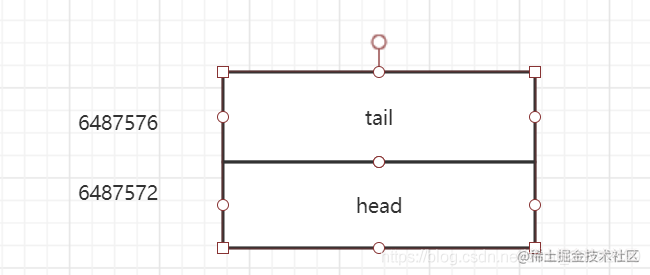当&(cat.tail)操作是，指针指向是这样的：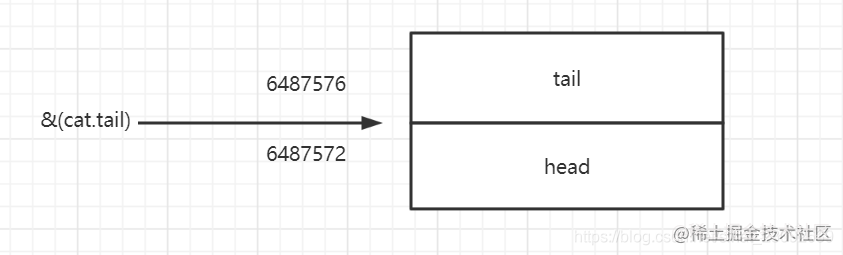关键点来了，当强转指针类型的时候，(Cat*)&(cat.tail)，计算机把Cat当做上方蓝色部分的：此时执行((Cat*)&(cat.tail))->head=12;，计算机已经把真正的tail当做head了，所以表面上是赋值给head，其实那是假的，真正的Cat是赋值给了tail，所以printf("cat.tail: %d\n", cat.tail);打印出来才是12。

``````int arr;
arr = 1 << 16;
**((short*)arr) = 2;**//关键，对arr指针进行强转为short类型，然后指针往前偏移6个步长
printf("arr: %d\n", arr);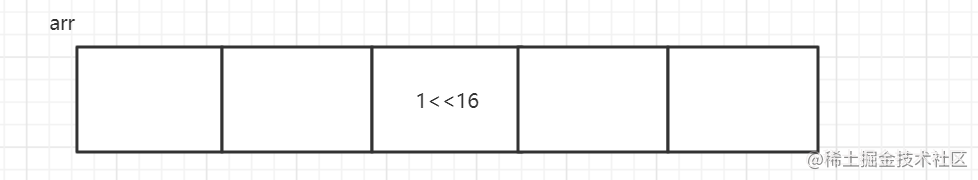而执行了((short*)arr) 指针强转之后，在计算机眼里，arr变成了：

（当前环境是小端模式）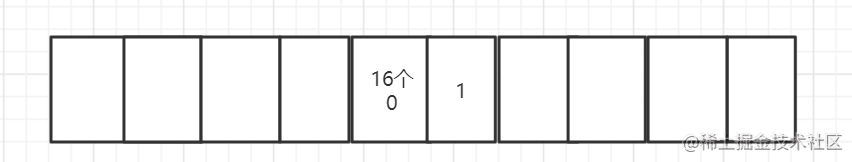执行了((short*)arr) = 2;之后：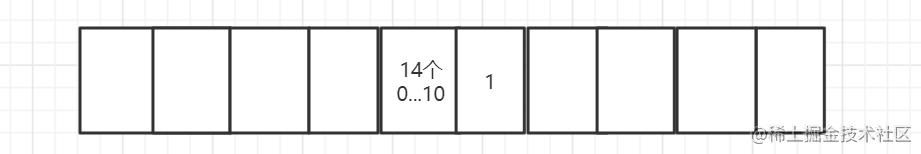执行printf("arr: %d\n", arr);的时候，上图的2个小格子又合并起来，得到结果为65538（小端模式，所以右边的小格子是高位）

``````int i = *(((short*)(&arr[-1]))+4);

Android